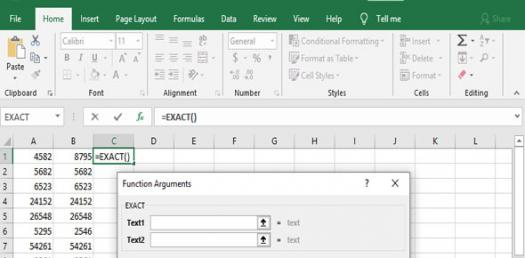# Excel Lesson 2-creating A Workbook

20 Questions | Attempts: 865
ShareSettingsThis quiz concerns Excel Lesson 2-Creating a workbook.

• 1.
Labels are aligned at the ________ edge of the cell.
• A.

Left

• B.

Right

• C.

Top

• D.

Bottom

• 2.
You can complete a cell entry by pressing
• A.

Enter

• B.

Tab

• C.

Shift+tab

• D.

An arrow key

• E.

Another cell

• 3.
The default font keyed in a new work book is.
• A.

12 pt. Times New Romans

• B.

10 pt. Arial

• C.

• D.

10 pt. Times New Roman

• 4.
True or False.  If you choose a larger font size, the height of the row is automatically made taller.
• 5.
Which button allows you to copy cell formats from one cell to another?
• A.

Enter

• B.

Autofit

• C.

Format painter

• D.

Esc

• 6.
A_______ is a group of cells that form a retangle on the screen.
• A.

Label

• B.

Workbook

• C.

Worksheet

• D.

Column

• E.

Range

• 7.
How do you know a range is active?
• A.

It is highlighted on the screen.

• B.

It has a marquee around it.

• C.

The data changes to Bold to let me know it's active

• D.

I'm psychic & I just Know!!

• 8.
True or False.  A range has an address called an range address.
• A.

True

• B.

False

• 9.
An example of a  range address.
• A.

A1:B3

• B.

A1;B3

• C.

A1-B3

• D.

A1=B3

• E.

A1*B3

• 10.
When you start to select a range of cells, you will see a thick white, cross shaped pointer called a ________________.
• A.

Cell selecter

• B.

Cell cross

• C.

Selection pointer

• D.

Range selecter

• 11.
If labels spill onto the column to it's right & the column on the right is not empty you need to ________________.
• A.

Resize the column

• B.

Complete your calculations by hand, excel is too hard to learn.

• C.

Select the column to delete it, you didn't need it anyway.

• D.

Leave it the way it is.

• E.

Widen it so the label is not cut off.

• 12.
A__________ is a single point of color on the screen.
• A.

Pixel

• B.

Cell

• C.

Border

• D.

Margin

• 13.
A value is always _____________ aligned in the cells & is included in calculations.
• A.

Left

• B.

Centered

• C.

Justified

• D.

Right

• 14.
This button allows you to incorporate the currency format to data.
• A.

.

• B.

%

• C.

=

• D.

-

• E.

\$

• 15.
When you key the date, excel applies a standard format that shows:
• A.

2 digits for the month, 2 digits for the day, & either 2 or 4 digits for the year.

• B.

Excel leaves it exactly the way I type it!

• C.

The formula to change the date.

• 16.
What does the function Sum do?
• A.

Adds the values in the range & then divides by the number of cells in the range.

• B.

Determines the largest value in a range.

• C.

Multiplies the values in a range.

• D.

Adds the values in the cell

• 17.
You can check results of your calculations by using:
• A.

Auto fit

• B.

Auto replace

• C.

Auto correct

• D.

Auto calculate

• 18.
A_________ is a built in mathematical formula included in excel.
• A.

Cell

• B.

Formula

• C.

Range

• D.

• E.

Function

• 19.
A________ is a series of instructions that performs a calculation & displays an answer.
• A.

Function

• B.

Formula

• C.

Range

• D.

Cell

• 20.
• A.

=

• B.

-

• C.

*

• D.

+

• E.

/

## Related TopicsBack to top
×

Wait!
Here's an interesting quiz for you.